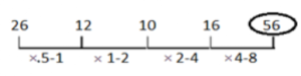# Number Series Questions

03 September 2023

## What is Number Series?

Number series is a sequence or orderly arrangement  formed with numbers. Number Series is the one of the most important topic of Mathematics. In the number series questions, the series are based on sequences of numbers that follow a logical pattern based on basic arithmetic concepts and we have to detect that concept then predict the next number in the sequence by following the same logic.

## Number Series Reasoning Questions With Answers

B) 11

C) 9

D) 14

A) 4

B) 11

C) 56

D) 5

A) 27

B) 25

C) 23

D) 29

A) 22

B) 41

C) 19

D) 23

### Q5) Look at this series: 2, 1, (1/2), (1/4), ... What number should come next?

A) (1/3)

B) (1/8)

C) (2/8)

D) (1/16)

#### Solution :

This is a simple division series; each number is one-half of the previous number. In other terms
to say, the number is divided by 2 successively to get the next result.
4/2 = 2
2/2 = 1
1/2 = 1/2
(1/2)/2 = 1/4
(1/4)/2 = 1/8 and so on.

### Q6) 26, 12, 10, 16, ?

A) 50

B) 52

C) 53

D) 56

#### Solution :### Q7) Look carefully for the pattern, and then choose which pair of numbers comes next.

2 44 4 41 6 38 8

A) 10 12

B) 35 32

C) 34 9

D) 35 10

#### Solution :

Here, there are two alternating patterns, one addition and one subtraction. The first starts with 2 and increases by 2; the second starts with 44 and decreases by 3.

### Q8) What should come in place of the question mark '?' in the following number series?

2916, 972, ?, 108, 36, 12

A) 324

B) 234

C) 248

D) 391

#### Solution :

2916 ÷ 3 = 972
972 ÷ 3 = 324 = ?
324 ÷ 3 = 108
108 ÷ 3 = 36
36 ÷ 3 = 12

### Q9) 4, 6, 9, 13,

A) 15

B) 12

C) 18

D) 17

#### Solution :

The difference between two consecutive numbers is increasing in the order of 2,3, 4, 5, etc.

### Q10) 210 209 213 186 202 (?)

A) 138

B) 77

C) 177

D) 327

#### Solution :

1. (2) The pattern of the number series is :
210 – 1³= 209
209 + 2² = 213
213 – 3³ = 186
186 + 4² = 202
202 – 5³ = 202 – 125 = 77